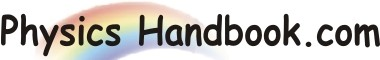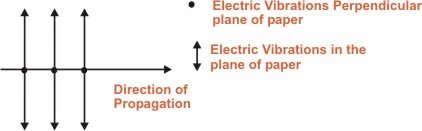HOME TOPICS DEFINITIONS TABLES LAWS INVENTIONS EXPERIMENTS QUIZ VIDEOS
 A B C D E F G H I J K L M N O P Q R S T U V W X Y Z
Unpolarised Light
Most sources of light emit unpolarised light i.e. vibrations take place in randomly oriented directions perpendicular to the travel of the wave.
When light is emitted by a source, it is as a result of electron transitions within the individual atoms of the source. These transitions occur rapidly and each gives rise to a wave for a short time.
Thus, the resultant light consists of many different waves originating at random times from atoms that are oriented randomly relative to each other. The amplitudes of vibrations are equal.
Unpolarised light consists of components of polarized in all possible directions perpendicular to the direction of propagation of the wave.Each of these polarization directions can be resolved into components along two mutually perpendicular directions. Thus, an unpolarised beam can be thought of as two plane polarized beams of equal magnitude perpendicular to one another. This give rise to the commonly used pictorial representation of unpolarised light wave shown in figure.
One beam is represented by dots and the other of equal magnitude by arrows. The dots represent vibrations perpendicular to the plane of the paper while arrows represents vibrations in the plane of the paper.